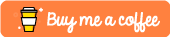# Geometry Kit

A set of utils that help with geometry (line, circle, triangle, polygon,...)

!!! Warning: This package is still in initial stage and no where near perfect. Still missing a lot. but it will be improve with time for sure. Any contribution is appreciated

## Features

• distance from a point to a line
• check if to line segments intersect
• find intersect point of 2 line segments
• get area or perimeter of shapes,
• calculate circumcircle or incircle of a polygon
• check if a point is in side a polygon

## Installing the library:

``````dependencies:
geometry_kit: <latest_version>
``````

Then import it wherever you want to use it:

``````import 'package:fetching_state/fetching_state.dart';
``````

## Usage

``````
// Line
final line1 = Line(Point(0, 2), Point(2, 0));
final line2 = Line(Point(0, -1), Point(3, 2));

final intersect = LineUtils.getSegmentIntersect(line1, line2);

print(intersect); //Point(1.5, 0.5)
``````
``````//Polygon
final polygon = [
Point(1, 0),
Point(0, 2),
Point(0, 3),
Point(2, 5),
Point(3, 5),
Point(5, 3),
Point(5, 1),
Point(3, 0),
];

final point1 = Point<num>(5, 2);
var isInside = PolygonUtils.isInsidePolygon(point1, polygon);
print(isInside); // true
``````

## Appreciate Your Feedbacks and Contributes

I'm not a math guy, to be honest, I'm stupid at math your feedbacks and contributes will be much appreciated.

If you find anything need to be improve or want to request a feature. Please go ahead and create an issue in the Github repo

geometry_kit